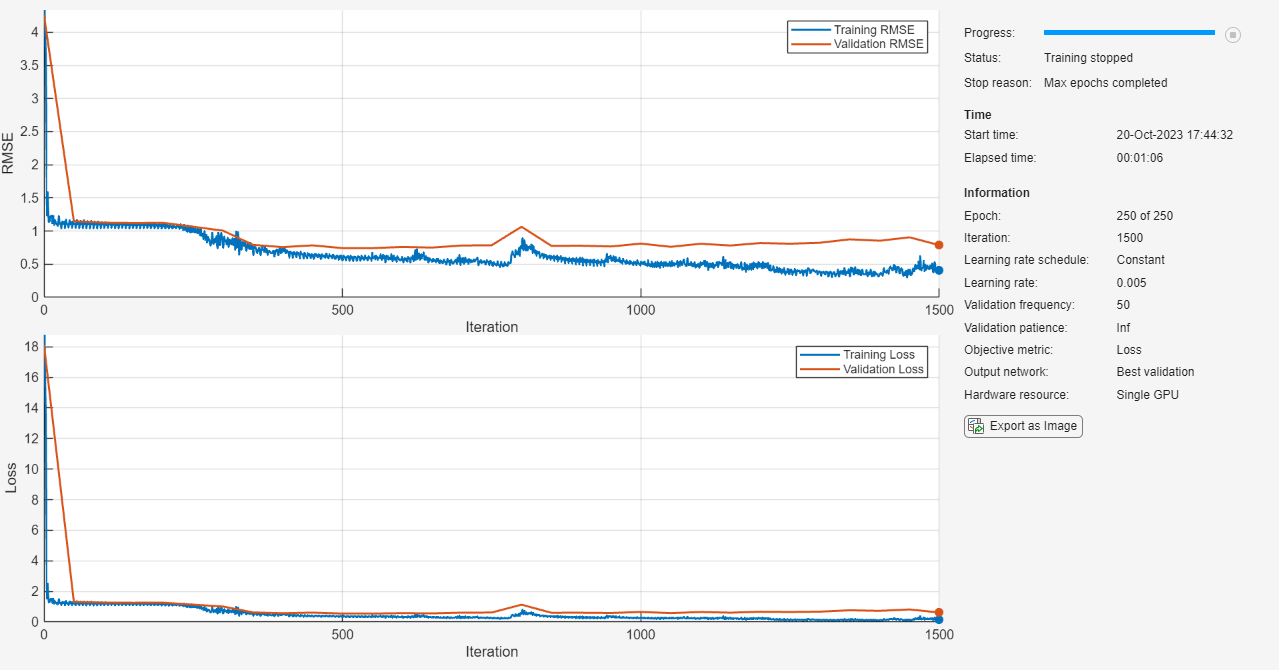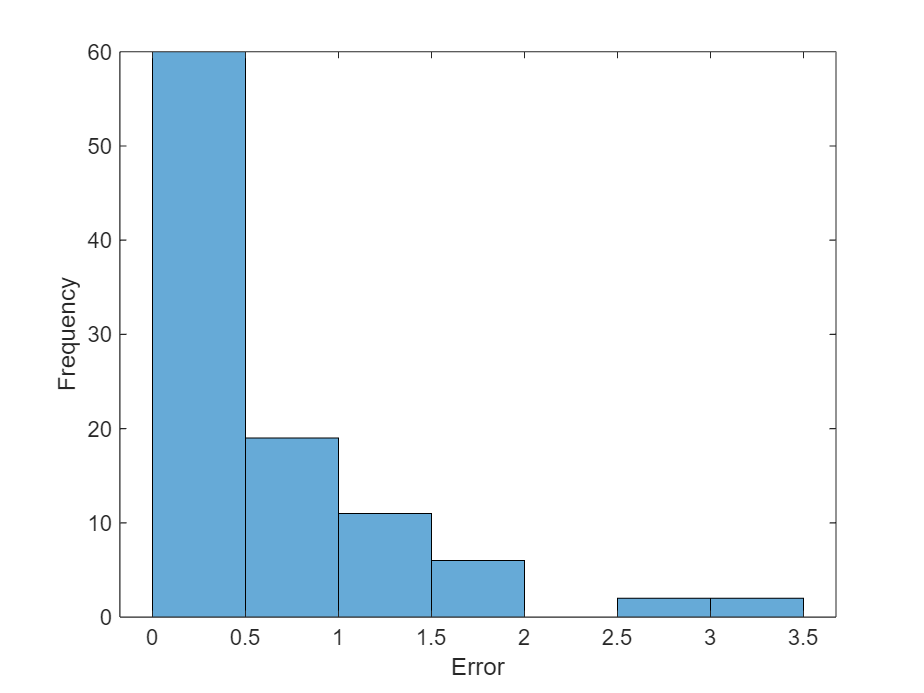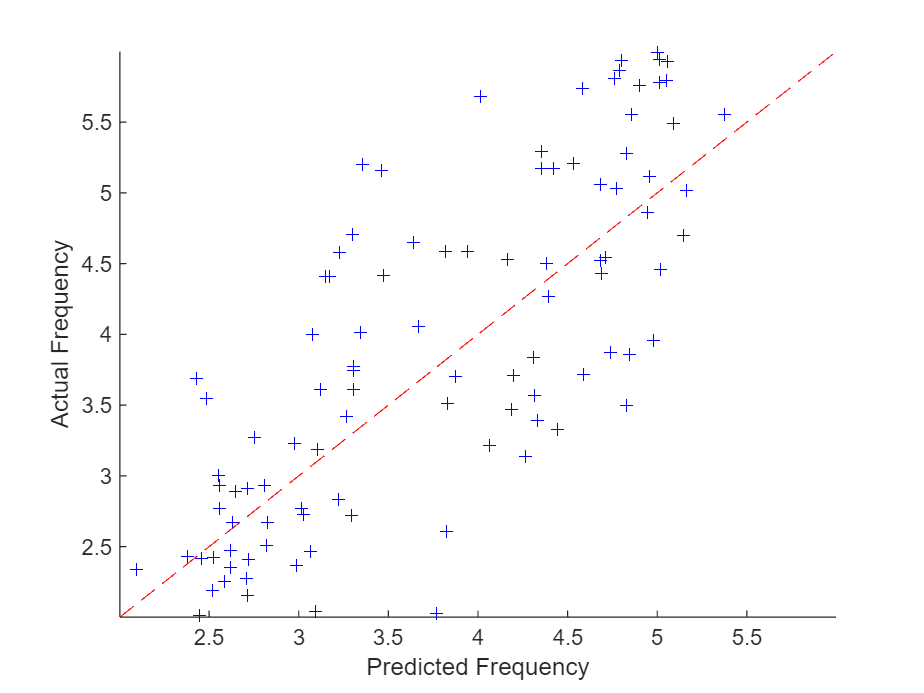# Sequence-to-One Regression Using Deep Learning

This example shows how to predict the frequency of a waveform using a long short-term memory (LSTM) neural network.

You can use an LSTM neural network to predict a numeric response of a sequence using a training set of sequences and target values. An LSTM network is a recurrent neural network (RNN) that processes input data by looping over time steps and updating the network state. The network state contains information remembered over previous time steps. Examples of numeric responses of a sequence include:

• Properties of the sequence, such as its frequency, maximum value, and mean.

• Values of past or future time steps of the sequence.

This example trains a sequence-to-one regression LSTM network using the Waveform data set, which contains 1000 synthetically generated waveforms of varying lengths with three channels. To determine the frequency of a waveform using conventional methods, see `fft`.

Load the example data from `WaveformData.mat`. The data is a `numObservations`-by-1 cell array of sequences, where `numObservations` is the number of sequences. Each sequence is a `numChannels`-by-`numTimeSteps` numeric array, where `numChannels` is the number of channels of the sequence and `numTimeSteps` is the number of time steps in the sequence. The corresponding targets are in a `numObservations`-by-`numResponses` numeric array of the frequencies of the waveforms, where `numResponses` is the number of channels of the targets.

`load WaveformData`

View the number of observations.

`numObservations = numel(data)`
```numObservations = 1000 ```

View the sizes of the first few sequences and the corresponding frequencies.

`data(1:4)`
```ans=4×1 cell array {3×103 double} {3×136 double} {3×140 double} {3×124 double} ```
`freq(1:4,:)`
```ans = 4×1 5.8922 2.2557 4.5250 4.4418 ```

View the number of channels of the sequences. For network training, each sequence must have the same number of channels.

`numChannels = size(data{1},1)`
```numChannels = 3 ```

View the number of responses (the number of channels of the targets).

`numResponses = size(freq,2)`
```numResponses = 1 ```

Visualize the first few sequences in plots.

```figure tiledlayout(2,2) for i = 1:4 nexttile stackedplot(data{i}', DisplayLabels="Channel " + (1:numChannels)) xlabel("Time Step") title("Frequency: " + freq(i)) end```### Prepare Data for Training

Set aside data for validation and testing. Partition the data into a training set containing 80% of the data, a validation set containing 10% of the data, and a test set containing the remaining 10% of the data.

```[idxTrain,idxValidation,idxTest] = trainingPartitions(numObservations, [0.8 0.1 0.1]); XTrain = data(idxTrain); XValidation = data(idxValidation); XTest = data(idxTest); TTrain = freq(idxTrain); TValidation = freq(idxValidation); TTest = freq(idxTest);```

### Define LSTM Network Architecture

Create an LSTM regression network.

• Use a sequence input layer with an input size that matches the number of channels of the input data.

• For a better fit and to prevent the training from diverging, set the `Normalization` option of the sequence input layer to "`zscore`". This normalizes the sequence data to have zero mean and unit variance.

• Use an LSTM layer with 100 hidden units. The number of hidden units determines how much information is learned by the layer. Larger values can yield more accurate results but can be more susceptible to overfitting to the training data.

• To output a single time step for each sequence, set the `OutputMode` option of the LSTM layer to "`last`".

• To specify the number of values to predict, include a fully connected layer with a size matching the number of predictors, followed by a regression layer.

```numHiddenUnits = 100; layers = [ ... sequenceInputLayer(numChannels, Normalization="zscore") lstmLayer(numHiddenUnits, OutputMode="last") fullyConnectedLayer(numResponses) regressionLayer]```
```layers = 4×1 Layer array with layers: 1 '' Sequence Input Sequence input with 3 dimensions 2 '' LSTM LSTM with 100 hidden units 3 '' Fully Connected 1 fully connected layer 4 '' Regression Output mean-squared-error ```

### Specify Training Options

Specify the training options.

• Train using the Adam optimizer.

• Train for 250 epochs. For larger data sets, you might not need to train for as many epochs for a good fit.

• Specify the sequences and responses used for validation.

• Output the network that gives the best, i.e. lowest, validation loss.

• Set the learning rate to 0.005.

• Truncate the sequences in each mini-batch to have the same length as the shortest sequence. Truncating the sequences ensures that no padding is added, at the cost of discarding data. For sequences where all of the time steps in the sequence are likely to contain important information, truncation can prevent the network from achieving a good fit.

• Display the training process in a plot.

• Disable the verbose output.

```options = trainingOptions("adam", ... MaxEpochs=250, ... ValidationData={XValidation TValidation}, ... OutputNetwork="best-validation-loss", ... InitialLearnRate=0.005, ... SequenceLength="shortest", ... Plots="training-progress", ... Verbose= false);```

### Train LSTM Network

Train the LSTM network with the specified training options using the `trainNetwork` function.

`net = trainNetwork(XTrain, TTrain, layers, options);`### Test LSTM Network

Make predictions using the test data.

`YTest = predict(net,XTest, SequenceLength="shortest");`

Visualize the first few predictions in a plot.

```figure tiledlayout(2,2) for i = 1:4 nexttile stackedplot(XTest{i}',DisplayLabels="Channel " + (1:numChannels)) xlabel("Time Step") title("Predicted Frequency: " + string(YTest(i))) end```Visualize the mean squared errors in a histogram.

```figure histogram(mean((TTest - YTest).^2,2)) xlabel("Error") ylabel("Frequency")```Calculate the overall root mean squared error.

`rmse = sqrt(mean((YTest-TTest).^2))`
```rmse = single 0.6865 ```

Plot the predicted frequencies against the actual frequencies.

```figure scatter(YTest,TTest, "b+"); xlabel("Predicted Frequency") ylabel("Actual Frequency") hold on m = min(freq); M=max(freq); xlim([m M]) ylim([m M]) plot([m M], [m M], "r--")```